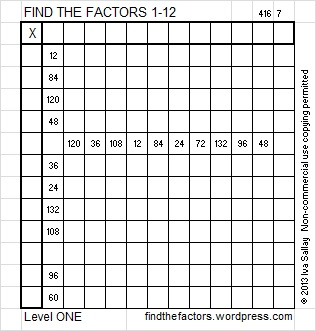# 96 and Level 1

Contents

### Today’s Puzzle:

Write the numbers from 1 to 12 in both the top row and the first column so that this puzzle will function like a multiplication table:This week’s puzzles and last week’s solutions: 12 Factors 2014-04-21

### Factors of 96:

96 is a composite number. 96 = 1 x 96, 2 x 48, 3 x 32, 4 x 24, 6 x 16, or 8 x 12. Factors of 96: 1, 2, 3, 4, 6, 8, 12, 16, 24, 32, 48, 96. Prime factorization: 96 = 2 x 2 x 2 x 2 x 2 x 3, which can also be written as 2⁵ x 3.Sometime 96 is a clue in the FIND THE FACTORS 1 – 12 puzzles. Even though it has many other factors, we only use 8 x 12 in the puzzles.

### Sum-Difference Puzzle:

6 has two factor pairs. One of those factor pairs adds up to 5 and the other one subtracts to 5. Can you place its factors in the correct boxes in the first puzzle?

96 has six factor pairs. One of those factor pairs adds up to 20, and the another subtracts to 20. If you can identify those two factor pairs, then you can solve the second puzzle!The second puzzle really is just the first puzzle in disguise. Why would I say that?

### Solution to This Week’s Puzzle:This site uses Akismet to reduce spam. Learn how your comment data is processed.